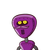# if two positive integers p and q are written as p= a²b³ and q=a³b ; a,b are prime numbers then verify:LCM (p,q) x HCF (p,q)=

if two positive integers p and q are written as p= a²b³ and q=a³b ; a,b are prime numbers then verify:
LCM (p,q) x HCF (p,q)= pq​

### 1 thought on “if two positive integers p and q are written as p= a²b³ and q=a³b ; a,b are prime numbers then verify:<br />LCM (p,q) x HCF (p,q)=”

1.Step-by-step explanation:

P= a^2b^3

Q= a^3b

Now,

Hcf = a^2b

LCM = a^3b^3

LCM *Hcf = a^2 *b *a^3*b^3

= a^2*b^3*a^3*b

=pq